Courses

# Worksheet Questions - Linear Equations in One Variable Class 8 Notes | EduRev

## Class 8 Mathematics by VP Classes

Created by: Vp Classes

## Class 8 : Worksheet Questions - Linear Equations in One Variable Class 8 Notes | EduRev

The document Worksheet Questions - Linear Equations in One Variable Class 8 Notes | EduRev is a part of the Class 8 Course Class 8 Mathematics by VP Classes.
All you need of Class 8 at this link: Class 8

Solve the following equations:

1. 2x – 3 = 7

2. 2z + 9 = 4

3.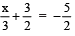4.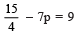5.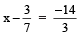6.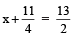7.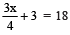8. 6.6x – 3.2 = 10

9. 8 – 3x = –10

10.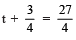1. x = 5

2. z = -5/2

3. x = –12

4. p = -3/4

5. x = -13/3

6. x = 15/4

7. x = 20

8. x = 34/33

9. x = 6

10. t = 6

Solve the following equations:

1. The sum of two numbers is 74. If one of the numbers is 10 more than the other, then what are the numbers?

2. What should be added to twice the rational number -7/3 to get 3/7 ?

3. The sum of three consecutive numbers is 57. Find the numbers.

4. The sum of three consecutive multiples of 11 is 363. Find the multiples.

5. Two whole numbers are in the ratio of 2 : 5. If their difference is 66, then find whole numbers.

6. The perimeter of a rectangle is 13 cm. If its width is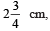then find its length.

7. The present age of Prabha’s mother is three times the present age of Prabha. After 5 years their ages will add to 66 years. Find their present ages.

8. Ramani has 3 times as many two-rupee coins as she has five-rupee coins. If she has in all a sum of Rs 77, then how many coins of each denomination does she have?

9. Kaveri has a total of Rs 590 as currency notes is the denominations of Rs 50, Rs 20 and Rs 10. The ratio of the number of Rs 50 notes and Rs 20 notes is 3 : 5. If she has a total of 25 notes, how many notes of each denomination she has?

10. A rational number is such that when you multiply it by 2/5 and add 2/3 to the product, you get 7/15. What is rational number?

1. 32 and 42

2. 107/21

3. 18, 19 and 20

4. 110, 121 and 132

5. 110 and 44

6.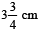7.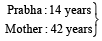8.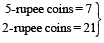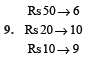10.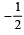Solve the following equations:

1. 2x – 3 = x + 2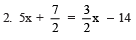3. 5x – 3 = 3x + 7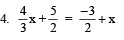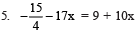1. x = 5

2. x = –5

3. x = 5

4. x = –12

5. x = -3/41

Solve for x:

Ques 1: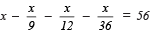Ques 2: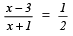Ques 3: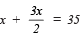Ques 4: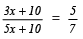Ques 5: x2 + 10x + 21 = x2 + 4x + 81

Ques 6: A number is such that it is as much greater than 45 as it is less than 75. Find the number.

Ques 7: A number is 20 greater than the sum of its one-third and one-ninth. Find the number.

Ques 8: Divide 40 into two parts such that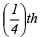of one part is equal to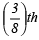of the other.

Ques 9: The numerator of a fraction is 2 less than its denominator. If 2 is subtracted from the numerator and 1 is the added to the denominator then the fraction becomes 1/2.

Find the fraction.

Ques 10: After 18 years I shall be 3 times as old as I was 4 years ago. Find my present age.

1. x = 72

2. x = 7

3. x = 14

4. x = 5

5. x = 10

6. 60

7. 36

8. 24 and 16

9. 7/9

10. 15 years

93 docs|16 tests

,

,

,

,

,

,

,

,

,

,

,

,

,

,

,

,

,

,

,

,

,

;